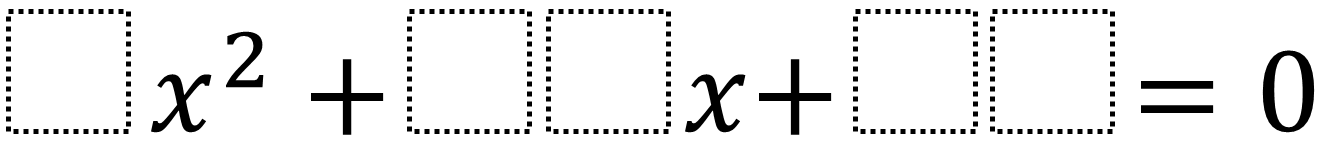Home > High School: Algebra > Factoring Quadratics 2 – One Solution

# Factoring Quadratics 2 – One Solution

Directions: Using the digits 0 to 9 at most one time each, fill in the boxes to so that there is only one integer solution. Note that you can use a 05 to make a 5.### Hint

How could you find the equation if you already knew the solutions?

The possible answers are: 4x^2 + 12x + 09, 4x^2 + 36x + 81, 9x^2 + 12x + 04, 9x^2 + 24x + 16, 9x^2 + 30x + 25, 9x^2 + 54x + 81

Source: Daniel Luevanos

## Solving Equations In Two Variables

Directions: Using the digits 1 to 9 at most one time each, fill in the …

1.•